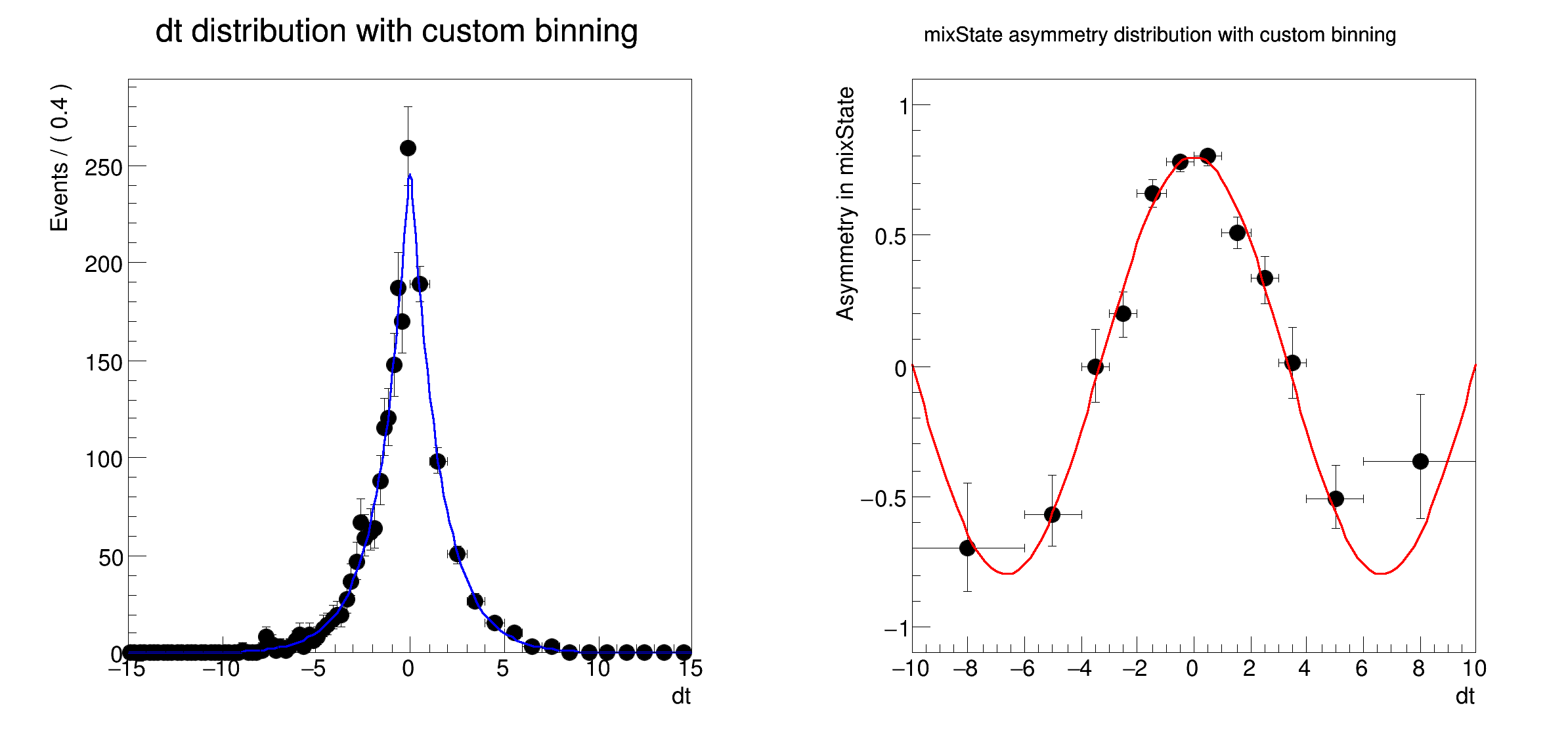ROOT   Reference Guiderf108_plotbinning.C File Reference

## Detailed DescriptionBasic functionality: plotting unbinned data with alternate and variable binnings␛[1mRooFit v3.60 -- Developed by Wouter Verkerke and David Kirkby␛[0m
Copyright (C) 2000-2013 NIKHEF, University of California & Stanford University
[#1] INFO:Plotting -- RooAbsReal::plotOn(bmix) plot on dt integrates over variables (mixState,tagFlav)
[#1] INFO:Plotting -- RooAbsReal::plotAsymOn(bmix) plot on dt projects variables (tagFlav)
#include "RooRealVar.h"
#include "RooDataSet.h"
#include "RooGaussModel.h"
#include "RooDecay.h"
#include "RooBMixDecay.h"
#include "RooCategory.h"
#include "RooBinning.h"
#include "RooPlot.h"
#include "TCanvas.h"
#include "TAxis.h"
#include "TH1.h"
using namespace RooFit;
{
// S e t u p m o d e l
// ---------------------
// Build a B decay pdf with mixing
RooRealVar dt("dt", "dt", -20, 20);
RooRealVar dm("dm", "dm", 0.472);
RooRealVar tau("tau", "tau", 1.547);
RooRealVar w("w", "mistag rate", 0.1);
RooRealVar dw("dw", "delta mistag rate", 0.);
RooCategory mixState("mixState", "B0/B0bar mixing state");
mixState.defineType("mixed", -1);
mixState.defineType("unmixed", 1);
RooCategory tagFlav("tagFlav", "Flavour of the tagged B0");
tagFlav.defineType("B0", 1);
tagFlav.defineType("B0bar", -1);
// Build a gaussian resolution model
RooRealVar bias1("bias1", "bias1", 0);
RooRealVar sigma1("sigma1", "sigma1", 0.1);
RooGaussModel gm1("gm1", "gauss model 1", dt, bias1, sigma1);
// Construct Bdecay (x) gauss
RooBMixDecay bmix("bmix", "decay", dt, mixState, tagFlav, tau, dm, w, dw, gm1, RooBMixDecay::DoubleSided);
// S a m p l e d a t a f r o m m o d e l
// --------------------------------------------
// Sample 2000 events in (dt,mixState,tagFlav) from bmix
RooDataSet *data = bmix.generate(RooArgSet(dt, mixState, tagFlav), 2000);
// S h o w d t d i s t r i b u t i o n w i t h c u s t o m b i n n i n g
// -------------------------------------------------------------------------------
// Make plot of dt distribution of data in range (-15,15) with fine binning for dt>0 and coarse binning for dt<0
// Create binning object with range (-15,15)
RooBinning tbins(-15, 15);
// Add 60 bins with uniform spacing in range (-15,0)
// Add 15 bins with uniform spacing in range (0,15)
// Make plot with specified binning
RooPlot *dtframe = dt.frame(Range(-15, 15), Title("dt distribution with custom binning"));
data->plotOn(dtframe, Binning(tbins));
bmix.plotOn(dtframe);
// NB: Note that bin density for each bin is adjusted to that of default frame binning as shown
// in Y axis label (100 bins --> Events/0.4*Xaxis-dim) so that all bins represent a consistent density distribution
// S h o w m i x s t a t e a s y m m e t r y w i t h c u s t o m b i n n i n g
// ------------------------------------------------------------------------------------
// Make plot of dt distribution of data asymmetry in 'mixState' with variable binning
// Create binning object with range (-10,10)
RooBinning abins(-10, 10);
// Add boundaries at 0, (-1,1), (-2,2), (-3,3), (-4,4) and (-6,6)
// Create plot frame in dt
RooPlot *aframe = dt.frame(Range(-10, 10), Title("mixState asymmetry distribution with custom binning"));
// Plot mixState asymmetry of data with specified custom binning
data->plotOn(aframe, Asymmetry(mixState), Binning(abins));
// Plot corresponding property of pdf
bmix.plotOn(aframe, Asymmetry(mixState));
// Adjust vertical range of plot to sensible values for an asymmetry
aframe->SetMinimum(-1.1);
aframe->SetMaximum(1.1);
// NB: For asymmetry distributions no density corrects are needed (and are thus not applied)
// Draw plots on canvas
TCanvas *c = new TCanvas("rf108_plotbinning", "rf108_plotbinning", 800, 400);
c->Divide(2);
c->cd(1);
dtframe->GetYaxis()->SetTitleOffset(1.6);
dtframe->Draw();
c->cd(2);
aframe->GetYaxis()->SetTitleOffset(1.6);
aframe->Draw();
}
#define c(i)
Definition: RSha256.hxx:101
virtual RooPlot * plotOn(RooPlot *frame, const RooCmdArg &arg1=RooCmdArg::none(), const RooCmdArg &arg2=RooCmdArg::none(), const RooCmdArg &arg3=RooCmdArg::none(), const RooCmdArg &arg4=RooCmdArg::none(), const RooCmdArg &arg5=RooCmdArg::none(), const RooCmdArg &arg6=RooCmdArg::none(), const RooCmdArg &arg7=RooCmdArg::none(), const RooCmdArg &arg8=RooCmdArg::none()) const
Definition: RooAbsData.cxx:547
RooArgSet is a container object that can hold multiple RooAbsArg objects.
Definition: RooArgSet.h:29
Class RooBMixDecay is a RooAbsAnaConvPdf implementation that describes the decay of B mesons with the...
Definition: RooBMixDecay.h:23
Class RooBinning is an implements RooAbsBinning in terms of an array of boundary values,...
Definition: RooBinning.h:28
RooCategory is an object to represent discrete states.
Definition: RooCategory.h:27
RooDataSet is a container class to hold unbinned data.
Definition: RooDataSet.h:33
Class RooGaussModel implements a RooResolutionModel that models a Gaussian distribution.
Definition: RooGaussModel.h:26
A RooPlot is a plot frame and a container for graphics objects within that frame.
Definition: RooPlot.h:44
virtual void SetMinimum(Double_t minimum=-1111)
Set minimum value of Y axis.
Definition: RooPlot.cxx:1091
TAxis * GetYaxis() const
Definition: RooPlot.cxx:1263
static RooPlot * frame(const RooAbsRealLValue &var, Double_t xmin, Double_t xmax, Int_t nBins)
Create a new frame for a given variable in x.
Definition: RooPlot.cxx:249
virtual void SetMaximum(Double_t maximum=-1111)
Set maximum value of Y axis.
Definition: RooPlot.cxx:1081
virtual void Draw(Option_t *options=0)
Draw this plot and all of the elements it contains.
Definition: RooPlot.cxx:691
RooRealVar represents a variable that can be changed from the outside.
Definition: RooRealVar.h:39
virtual void SetTitleOffset(Float_t offset=1)
Set distance between the axis and the axis title.
Definition: TAttAxis.cxx:293
The Canvas class.
Definition: TCanvas.h:23
RooCmdArg Binning(const RooAbsBinning &binning)
RooCmdArg Asymmetry(const RooCategory &cat)
The namespace RooFit contains mostly switches that change the behaviour of functions of PDFs (or othe...
const char * Title
Definition: TXMLSetup.cxx:68
Ta Range(0, 0, 1, 1)
Date
July 2008

Definition in file rf108_plotbinning.C.